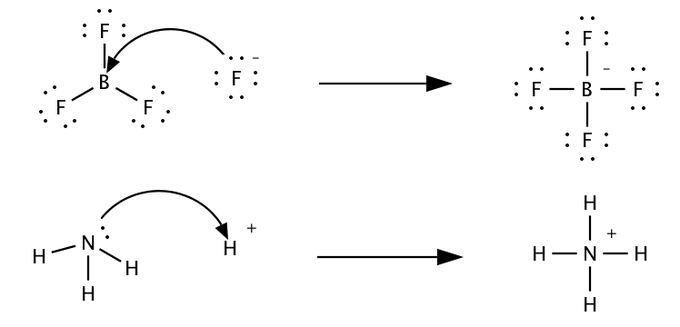## Lewis Acid and Base Molecules

#### Learning Objective

• Recognize Lewis acids and bases in chemical reactions.

#### Key Points

• A Lewis acid is an electron-pair acceptor; a Lewis base is an electron-pair donor.
• Some molecules can act as either Lewis acids or Lewis bases; the difference is context-specific and varies based on the reaction.
• Lewis acids and bases result in the formation of an adduct rather than a simple displacement reaction, as with classical acids and bases. An example is HCl vs H+: HCl is a classical acid, but not a Lewis acid; H+ is a Lewis acid when it forms an adduct with a Lewis base.

#### Terms

• covalent bonda chemical bond in which two atoms are connected to each other by sharing two or more electrons
• nucleophileliterally “lover of nuclei,” Lewis bases are often referred to as this because they seek to donate their electron pairs to electron-poor species, such as H+

A Lewis acid is defined as an electron-pair acceptor, whereas a Lewis base is an electron-pair donor. Under this definition, we need not define an acid as a compound that is capable of donating a proton, because under the Lewis definition, H+ itself is the Lewis acid; this is because, with no electrons, H+ can accept an electron pair.

A Lewis base, therefore, is any species that donates a pair of electrons to a Lewis acid. The “neutralization” reaction is one in which a covalent bond forms between an electron-rich species (the Lewis base) and an electron-poor species (the Lewis acid). For this reason, Lewis bases are often referred to as nucleophiles (literally, “lovers of nuclei”), and Lewis acids are sometimes called electrophiles (“lovers of electrons”). This definition is useful because it not only covers all the acid-base chemistry with which we are already familiar, but it describes reactions that cannot be modeled by Arrhenius or Bronsted-Lowry acid-base chemistry. For now however, we will consider how the Lewis definition applies to classic acid-base neutralization.

## Applying the Lewis Definition to Classical Acid-Base Chemistry

Consider the familiar reaction of NaOH and HCl:

$NaOH(aq)+HCl(aq)\rightarrow NaCl(aq)+H_2O(l)$

We have previously described this as an acid-base neutralization reaction in which water and a salt are formed. This is still completely correct, but the Lewis definition describes the chemistry from a slightly different perspective. When considering Lewis acids and bases, the only real reaction of interest is the net ionic reaction:

$OH^-(aq)+H^+(aq)\rightarrow H_2O(l)$

Under the Lewis definition, hydroxide acts as the Lewis base, donating its electron pair to H+. Thus, in this version of the neutralization reaction, what interests us is not the salt that forms, but the covalent bond that forms between OH and H+ to form water. A significant hallmark for Lewis acid-base reactions is the formation of such a covalent bond between the two reacting species. The reaction’s final product is known as an adduct, because it forms from the addition of the Lewis base to the Lewis acid.Lewis acids and basesLewis acids (BF3, top, and H+, bottom) react with Lewis bases (F–, top, NH3, bottom) to form products known as adducts. Note that the first reaction cannot be described by Arrhenius or Bronsted-Lowry acid-base chemistry.

## Beyond Classical Acid-Base Chemistry

By treating acid-base reactions in terms of electron pairs instead of specific substances, the Lewis definition can apply to reactions that do not fall under other definitions of acid-base reactions. For example, a silver cation behaves as a Lewis acid with respect to ammonia, which behaves as a Lewis base, in the following reaction:

$Ag^+(aq) + 2\;NH_3 \rightarrow [Ag(NH_3)_2]^+$

This reaction results in the formation of diamminesilver(I), a complex ion; it is perfectly described by Lewis acid-base chemistry, but is unclassifiable according to more traditional Arrhenius and Bronsted-Lowry definitions.

## Application to Organic Chemistry

In organic chemistry, it is useful to understand that nucleophiles are Lewis bases and electrophiles are Lewis acids. Nearly all reactions in organic chemistry can be considered Lewis acid-base processes.